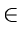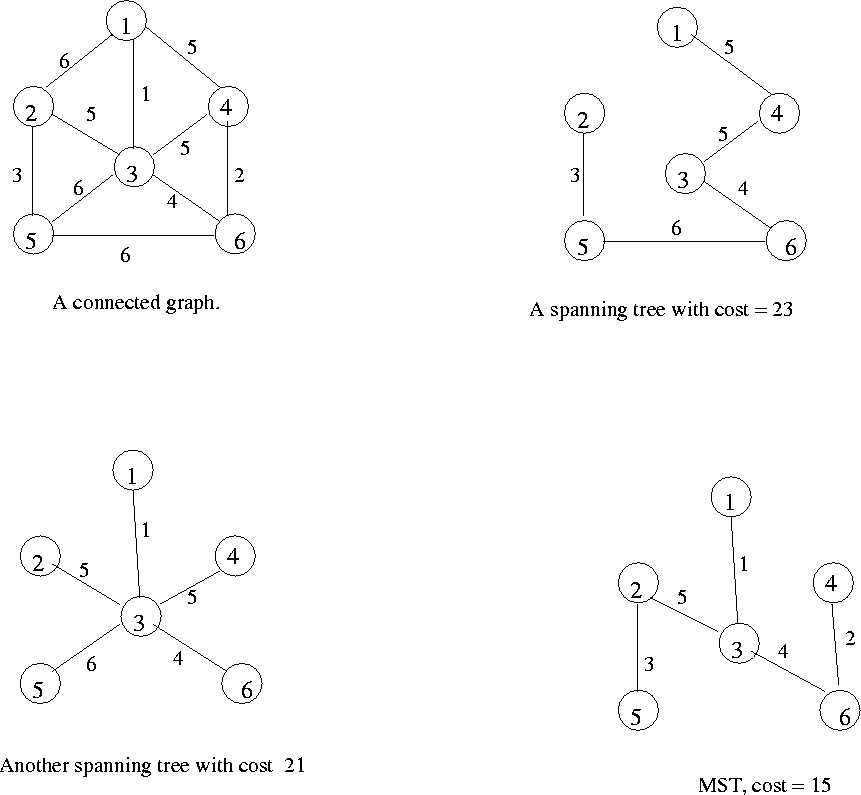Next:8.3.1 MST PropertyUp:8. Undirected GraphsPrevious:8.2.1 Breadth-first search of undirected graph

# 8.3 Minimum-Cost Spanning Trees

Let G = (V, E) be a connected graph in which each edge (u, vE has an associated cost C(u, v).
• A Spanning Tree for G is a subgraph of G that it is a free tree connecting all vertices in V. The cost of a spanning tree is the sum of costs on its edges.
• An MST of G is a spanning tree of G having a minimum cost.
• See Figure 8.4 for several examples.eEL,CSA_Dept,IISc,Bangalore